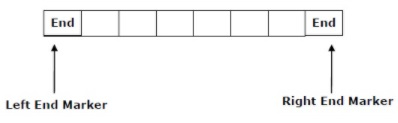# What is Linear bounded automata in TOC?

A deterministic linear bounded automaton (LBA) is 9-tuple automata

G = ( Q, Σ, E, δ, ε, q0, GL, GR, F)

Where,

• Q is set of all states

• Σ is set of all terminals

• E input alphabet.

• δ is set of transitions

• q0 initial state

• GL left bound of tape

• GR right bound of tape.

• F set of final state

A linear bounded automaton is called a multi-track non-deterministic Turing machine which has a tape of some bounded finite length.

Length = function (Length of the initial input string, constant c)

The computation in linear bounded automata is restricted to the constant bounded area. The input alphabet here contains two special symbols that serve as left end markers and right end markers. It explains that the transitions neither move to the left of the left end marker nor to the right of the right end marker of the tape.

A deterministic linear bounded automaton is context-sensitive and the linear bounded automaton with empty language is undecidable.## Problem

How linear bounded automata is constructed to accept the language L={an|n=m2,m>=1}?

## Solution

For the given language L={an|n=m2,m>=1}, the strings it is generated is −

L(G) ={a.aa.ab.aaa.aab.abb…….}

• S aA, A,aA,A,B,B,bB,B

• S aA, aB, a, a (accepted)

• S aA, aaA aaB, aa, aa (accepted)

• S aA, aB abB, ab, ab (accepted)

• SaA, aaA, aaaA, aaa aaa (accepted)

• SaA, aaA, aaB, aabB aab, aab (accepted)

• SaA, aB, abB, abbB, abb, abb (accepted)

We can prove every single string in L(G) here is accepted by the language by the production set.

G={{S,AB};{a,b}S, {S aA, A,aA/B, B/bB}}.